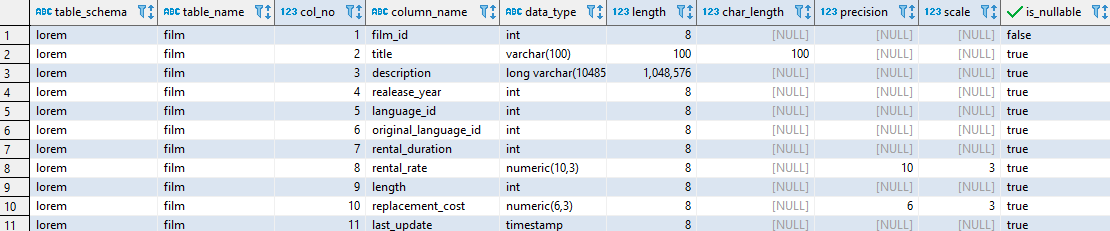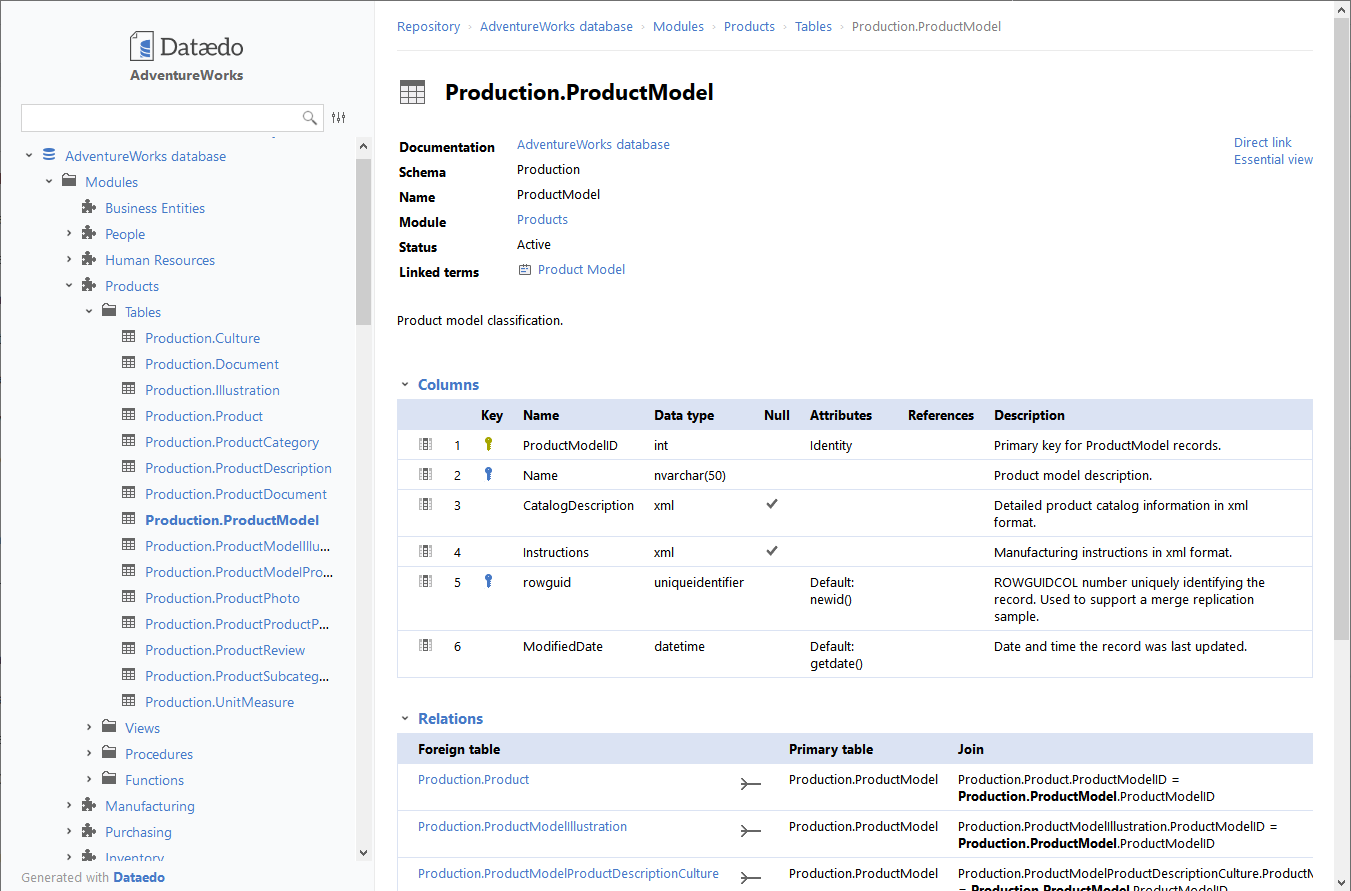## List all columns in specific table in Vertica database

Article for:

Query below returns a list of all columns in a specific table in PostgreSQL.

## Query

``````select table_schema,
table_name,
ordinal_position as col_no,
column_name,
data_type,
data_type_length as length,
character_maximum_length as char_length,
case when numeric_precision is null
then datetime_precision
else numeric_precision end as precision,
numeric_scale as scale,
is_nullable
from v_catalog.columns
where table_name = 'table_name' -- put table name here
-- and table_schema = 'schema_name'
order by table_schema,
table_name,
col_no;
``````

## Columns

• table_schema - schema name
• table_name - table name
• col_no - number of column in the table
• column_name - name of column
• data_type - column data type
• length - data type max length
• char_length - character datatype maximum length in characters
• precision - for numeric data type numeric precision and for timestamp data type time precision
• scale - numeric scale
• is_nullable - indicate if column can be null or not

## Rows

• One row represents one column
• Scope of rows: all columns in provided table
• Ordered by schema name, table name, column position in table

## Sample results## You could also get this

Get this interactive HTML data dictionary in minutes with Dataedo.See live HTML data dictionary sample